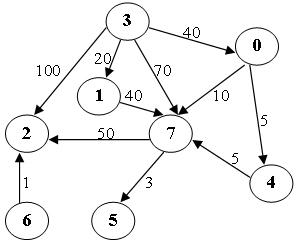# 6-17 Shortest Path  (25分)

Write a program to find the weighted shortest distances from any vertex to a given source vertex in a digraph. If there is more than one minimum path from v to w, a path with the fewest number of edges is chosen. It is guaranteed that all the weights are positive and such a path is unique for any vertex.

## Format of functions:

void ShortestDist( MGraph Graph, int dist[], int path[], Vertex S );


where MGraph is defined as the following:

typedef struct GNode *PtrToGNode;
struct GNode{
int Nv;
int Ne;
WeightType G[MaxVertexNum][MaxVertexNum];
};
typedef PtrToGNode MGraph;


The shortest distance from V to the source S is supposed to be stored in dist[V]. If V cannot be reached from S, store -1 instead. If W is the vertex being visited right before V along the shortest path from S to V, then path[V]=W. If V cannot be reached from S, path[V]=-1, and we have path[S]=-1.

## Sample program of judge:

#include <stdio.h>
#include <stdlib.h>

typedef enum {false, true} bool;
#define INFINITY 1000000
#define MaxVertexNum 10  /* maximum number of vertices */
typedef int Vertex;      /* vertices are numbered from 0 to MaxVertexNum-1 */
typedef int WeightType;

typedef struct GNode *PtrToGNode;
struct GNode{
int Nv;
int Ne;
WeightType G[MaxVertexNum][MaxVertexNum];
};
typedef PtrToGNode MGraph;

MGraph ReadG(); /* details omitted */

void ShortestDist( MGraph Graph, int dist[], int path[], Vertex S );

int main()
{
int dist[MaxVertexNum], path[MaxVertexNum];
Vertex S, V;

scanf("%d", &S);
ShortestDist( G, dist, path, S );

for ( V=0; V<G->Nv; V++ )
printf("%d ", dist[V]);
printf("\n");
for ( V=0; V<G->Nv; V++ )
printf("%d ", path[V]);
printf("\n");

return 0;
}

/* Your function will be put here */


## Sample Input (for the graph shown in the figure):8 11
0 4 5
0 7 10
1 7 40
3 0 40
3 1 20
3 2 100
3 7 70
4 7 5
6 2 1
7 5 3
7 2 50
3


## Sample Output:

40 20 100 0 45 53 -1 50
3 3 3 -1 0 7 -1 0


## 源码：

void ShortestDist( MGraph Graph, int dist[], int path[], Vertex S )
{
int i;
int visited[MaxVertexNum];
for(i=0;i<Graph->Nv;i++)
{
dist[i]=INFINITY;
path[i]=-1;
visited[i]=0;
if(i==S)
{
dist[i]=0;
}
}
while(1)
{
int minindex;
int min=INFINITY;
for(i=0;i<Graph->Nv;i++)
{
if(!visited[i]&&dist[i]<min)
{
minindex=i;
min=dist[minindex];
}
}
if(min==INFINITY)break;
visited[minindex]=1;
int j;
for(j=0;j<Graph->Nv;j++)
{
if(!visited[j]&&Graph->G[minindex][j]!=INFINITY)
{
if(dist[minindex]+Graph->G[minindex][j]<dist[j])
{
dist[j]=dist[minindex]+Graph->G[minindex][j];
path[j]=minindex;
}
}
}
}
for(i=0;i<Graph->Nv;i++)
if(dist[i]==INFINITY)dist[i]=-1;
}


©️2019 CSDN 皮肤主题: 黑客帝国 设计师: 上身试试